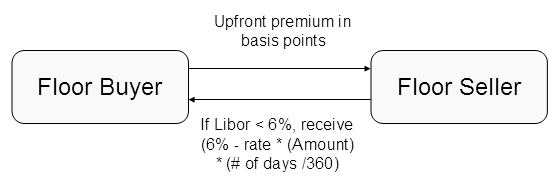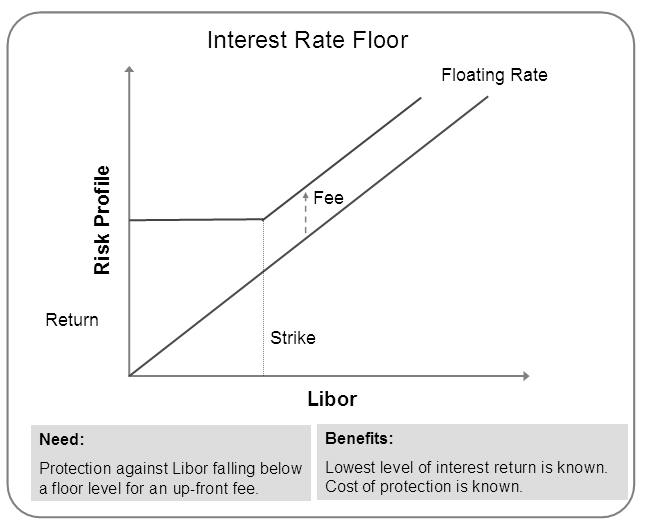# How Interest Rate Floors Work?

An interest rate floor is similar to an interest rate cap agreement. An interest rate floor is an agreement between the seller or provider of the floor and an investor which guarantees that the investor’s floating rate of return will not fall below a specified level over an agreed period of time. Analytically, this represents the purchase of a series of put options on the relevant interest index coinciding with the rollover dates of the investor’s floating rate assets.Similar to an interest rate cap, the investor selects a reference rate to hedge, the period of time to hedge and the level of protection desired in return for an up-front fee or premium.

If market rates fall below the floor rate, then the floor provider will make payments are made and the investor enjoys market rates of return.

The payoff of a floor is given by the following formula:

(Strike Rate-Index Level) x(# Days in period/360) x (Notional Amount)

For example suppose a floor had a strike of  6% based on a 3 month Libor, a notional amount of $10,000,00 and the number of days in the period was 90. If at reset date(day 90) the 3 month Libor was at 7%,then the floor provider would make no payments. If at reset date(date 90) the 3 month Libor was at 5%,then the floor provider would make a payment equal to : (6%-5%) x (90/360) x ($10,000,000) = \$250,000

Because the payer pays up an up-front fee, no additional obligations exist from the buyer so there is no residual credit risk.

The following data graphically depicts an interest rate floor.